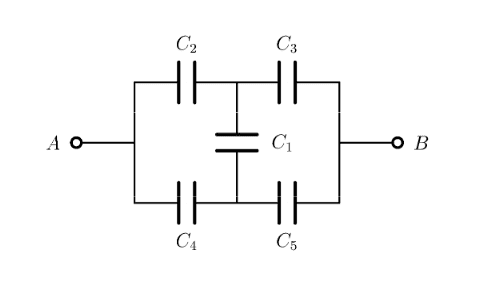# Potential difference across capacitor

Member advised to use the homework template for posts in the homework sections of PF.
Hi, the solution of my exercise says that the potential difference in the capacitor C1 is zero.Can you please explain me why it's the case ?

Thank you.

scottdave
Homework Helper
You don't have any values for those capacitors. This is an example of a bridge circuit. Read this article for some insight. https://en.wikipedia.org/wiki/Bridge_circuit

Resistors and capacitors work differently, but be assured that there are capacitor values which would result in the voltage at the top of the C1 to be the same voltage as the bottom of C1. For example, if we have 10.5 volts at the top and 10.5 volts at the bottom, then 10.5-10.5 = 0.

Owwww I'm sorry, I forgot to give the values !!

In fact, we have C2=C4 = 1.0μF and C3=C5 = 2.0μF.

However what if, in this case, we have C2 =/= C4 OR C3 =/=C5, C1 wouldn't be equal to zero anymore, right ?

berkeman
Mentor
However what if, in this case, we have C2 =/= C4 OR C3 =/=C5, C1 wouldn't be equal to zero anymore, right ?
Correct.

scottdave